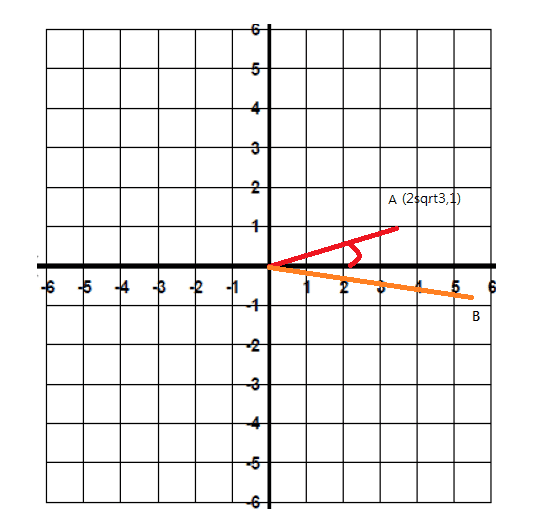# Rotation of complex number

Given A(2√3,1) in R^2 , rotate OA by 30° in clockwise direction and stretch the resulting vector by a factor of 6 to OB. Determine the coordinates of B in surd form using complex number technique.

i try to rewrite in Euler's form and I found the modulus was √13 but the argument could not be represented in radian, so i feel confuse to cope with this question.MENTOR Note: moved here from Linear Algebra hence no template

Last edited by a moderator:

jedishrfu
Mentor
Did you try drawing it to see where you went wrong?

Did you try drawing it to see where you went wrong?

yes, i draw the it in 2d plane with real and img. axis and roughly get the resulting vector, but still confuse with getting the exact number of the argument

jedishrfu
Mentor
Can you show us what you did step by step?

Can you show us what you did step by step?the above is the roughly work. I found the angle(argument) between OA and x-axis (real axis) was tan^-1(1/2√3)≈16.10211375
After rotate 30° clockwise, the angle between OB and x-axis was 16.10211375-30≈-13.89788625
and here i got trouble with the representation in surd form.
OA = 2√3+i =√13(cos16.10211375+isin16.10211375)
I found that complex number multiplication represent scaling and rotation, but my notes didnt contain explanation of this part

jedishrfu
Mentor
So the rotated number is sqrt(13)*(cos(-13.89) + I sin(-13.89) ) right?

Then take the negative out of the sin and cos to get it into better form.

Next you have to make it 6 times bigger.

Ray Vickson
Homework Helper
Dearly Missed
Given A(2√3,1) in R^2 , rotate OA by 30° in clockwise direction and stretch the resulting vector by a factor of 6 to OB. Determine the coordinates of B in surd form using complex number technique.

i try to rewrite in Euler's form and I found the modulus was √13 but the argument could not be represented in radian, so i feel confuse to cope with this question.MENTOR Note: moved here from Linear Algebra hence no template

The argument can be expressed in radians, but you need not bother doing that. Just express the clockwise 30° rotation as multiplication by a complex number of the form a + bi and then carry out the operations of standard complex-number multiplication. Your final answer need not involve any approximate decimal numbers, but can be expressed exactly in terms of square roots and the like.

•AlexChan and jedishrfu